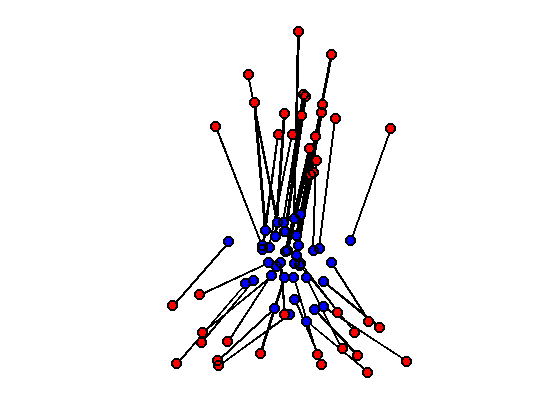$\newcommand{\NN}{\mathbb{N}} \newcommand{\CC}{\mathbb{C}} \newcommand{\GG}{\mathbb{G}} \newcommand{\LL}{\mathbb{L}} \newcommand{\PP}{\mathbb{P}} \newcommand{\QQ}{\mathbb{Q}} \newcommand{\RR}{\mathbb{R}} \newcommand{\VV}{\mathbb{V}} \newcommand{\ZZ}{\mathbb{Z}} \newcommand{\FF}{\mathbb{F}} \newcommand{\KK}{\mathbb{K}} \newcommand{\UU}{\mathbb{U}} \newcommand{\EE}{\mathbb{E}} \newcommand{\Aa}{\mathcal{A}} \newcommand{\Bb}{\mathcal{B}} \newcommand{\Cc}{\mathcal{C}} \newcommand{\Dd}{\mathcal{D}} \newcommand{\Ee}{\mathcal{E}} \newcommand{\Ff}{\mathcal{F}} \newcommand{\Gg}{\mathcal{G}} \newcommand{\Hh}{\mathcal{H}} \newcommand{\Ii}{\mathcal{I}} \newcommand{\Jj}{\mathcal{J}} \newcommand{\Kk}{\mathcal{K}} \newcommand{\Ll}{\mathcal{L}} \newcommand{\Mm}{\mathcal{M}} \newcommand{\Nn}{\mathcal{N}} \newcommand{\Oo}{\mathcal{O}} \newcommand{\Pp}{\mathcal{P}} \newcommand{\Qq}{\mathcal{Q}} \newcommand{\Rr}{\mathcal{R}} \newcommand{\Ss}{\mathcal{S}} \newcommand{\Tt}{\mathcal{T}} \newcommand{\Uu}{\mathcal{U}} \newcommand{\Vv}{\mathcal{V}} \newcommand{\Ww}{\mathcal{W}} \newcommand{\Xx}{\mathcal{X}} \newcommand{\Yy}{\mathcal{Y}} \newcommand{\Zz}{\mathcal{Z}} \newcommand{\al}{\alpha} \newcommand{\la}{\lambda} \newcommand{\ga}{\gamma} \newcommand{\Ga}{\Gamma} \newcommand{\La}{\Lambda} \newcommand{\Si}{\Sigma} \newcommand{\si}{\sigma} \newcommand{\be}{\beta} \newcommand{\de}{\delta} \newcommand{\De}{\Delta} \renewcommand{\phi}{\varphi} \renewcommand{\th}{\theta} \newcommand{\om}{\omega} \newcommand{\Om}{\Omega} \renewcommand{\epsilon}{\varepsilon} \newcommand{\Calpha}{\mathrm{C}^\al} \newcommand{\Cbeta}{\mathrm{C}^\be} \newcommand{\Cal}{\text{C}^\al} \newcommand{\Cdeux}{\text{C}^{2}} \newcommand{\Cun}{\text{C}^{1}} \newcommand{\Calt}{\text{C}^{#1}} \newcommand{\lun}{\ell^1} \newcommand{\ldeux}{\ell^2} \newcommand{\linf}{\ell^\infty} \newcommand{\ldeuxj}{{\ldeux_j}} \newcommand{\Lun}{\text{\upshape L}^1} \newcommand{\Ldeux}{\text{\upshape L}^2} \newcommand{\Lp}{\text{\upshape L}^p} \newcommand{\Lq}{\text{\upshape L}^q} \newcommand{\Linf}{\text{\upshape L}^\infty} \newcommand{\lzero}{\ell^0} \newcommand{\lp}{\ell^p} \renewcommand{\d}{\ins{d}} \newcommand{\Grad}{\text{Grad}} \newcommand{\grad}{\text{grad}} \renewcommand{\div}{\text{div}} \newcommand{\diag}{\text{diag}} \newcommand{\pd}{ \frac{ \partial #1}{\partial #2} } \newcommand{\pdd}{ \frac{ \partial^2 #1}{\partial #2^2} } \newcommand{\dotp}{\langle #1,\,#2\rangle} \newcommand{\norm}{|\!| #1 |\!|} \newcommand{\normi}{\norm{#1}_{\infty}} \newcommand{\normu}{\norm{#1}_{1}} \newcommand{\normz}{\norm{#1}_{0}} \newcommand{\abs}{\vert #1 \vert} \newcommand{\argmin}{\text{argmin}} \newcommand{\argmax}{\text{argmax}} \newcommand{\uargmin}{\underset{#1}{\argmin}\;} \newcommand{\uargmax}{\underset{#1}{\argmax}\;} \newcommand{\umin}{\underset{#1}{\min}\;} \newcommand{\umax}{\underset{#1}{\max}\;} \newcommand{\pa}{\left( #1 \right)} \newcommand{\choice}{ \left\{ \begin{array}{l} #1 \end{array} \right. } \newcommand{\enscond}{ \left\{ #1 \;:\; #2 \right\} } \newcommand{\qandq}{ \quad \text{and} \quad } \newcommand{\qqandqq}{ \qquad \text{and} \qquad } \newcommand{\qifq}{ \quad \text{if} \quad } \newcommand{\qqifqq}{ \qquad \text{if} \qquad } \newcommand{\qwhereq}{ \quad \text{where} \quad } \newcommand{\qqwhereqq}{ \qquad \text{where} \qquad } \newcommand{\qwithq}{ \quad \text{with} \quad } \newcommand{\qqwithqq}{ \qquad \text{with} \qquad } \newcommand{\qforq}{ \quad \text{for} \quad } \newcommand{\qqforqq}{ \qquad \text{for} \qquad } \newcommand{\qqsinceqq}{ \qquad \text{since} \qquad } \newcommand{\qsinceq}{ \quad \text{since} \quad } \newcommand{\qarrq}{\quad\Longrightarrow\quad} \newcommand{\qqarrqq}{\quad\Longrightarrow\quad} \newcommand{\qiffq}{\quad\Longleftrightarrow\quad} \newcommand{\qqiffqq}{\qquad\Longleftrightarrow\qquad} \newcommand{\qsubjq}{ \quad \text{subject to} \quad } \newcommand{\qqsubjqq}{ \qquad \text{subject to} \qquad }$

Optimal Transport with Linear Programming

# Optimal Transport with Linear Programming

This numerical tours details how to solve the discrete optimal transport problem (in the case of measures that are sums of Diracs) using linear programming.

## Installing toolboxes and setting up the path.

You need to download the following files: signal toolbox and general toolbox.

You need to unzip these toolboxes in your working directory, so that you have toolbox_signal and toolbox_general in your directory.

For Scilab user: you must replace the Matlab comment '%' by its Scilab counterpart '//'.

Recommandation: You should create a text file named for instance numericaltour.sce (in Scilab) or numericaltour.m (in Matlab) to write all the Scilab/Matlab command you want to execute. Then, simply run exec('numericaltour.sce'); (in Scilab) or numericaltour; (in Matlab) to run the commands.

Execute this line only if you are using Matlab.

getd = @(p)path(p,path); % scilab users must *not* execute this


Then you can add the toolboxes to the path.

getd('toolbox_signal/');
getd('toolbox_general/');


## Optimal Transport of Discrete Distribution

We consider two dicretes distributions $\forall k=0,1, \quad \mu_k = \sum_{i=1}^{n_k} p_{k,i} \de_{x_{k,i}}$ where $$n_0,n_1$$ are the number of points, $$\de_x$$ is the Dirac at location $$x \in \RR^d$$, and $$X_k = ( x_{k,i} )_{i=1}^{n_i} \subset \RR^d$$ for $$k=0,1$$ are two point clouds.

We define the set of couplings between $$\mu_0,\mu_1$$ as $\Pp = \enscond{ (\ga_{i,j})_{i,j} \in (\RR^+)^{n_0 \times n_1} }{ \forall i, \sum_j \ga_{i,j} = p_{0,i}, \: \forall j, \sum_i \ga_{i,j} = p_{1,j} }$

The Kantorovitch formulation of the optimal transport reads $\ga^\star \in \uargmin{\ga \in \Pp} \sum_{i,j} \ga_{i,j} C_{i,j}$ where $$C_{i,j} \geq 0$$ is the cost of moving some mass from $$x_{0,i}$$ to $$x_{1,j}$$.

The optimal coupling $$\ga^\star$$ can be shown to be a sparse matrix with less than $$n_0+n_1-1$$ non zero entries. An entry $$\ga_{i,j}^\star \neq 0$$ should be understood as a link between $$x_{0,i}$$ and $$x_{1,j}$$ where an amount of mass equal to $$\ga_{i,j}^\star$$ is transfered.

In the following, we concentrate on the $$L^2$$ Wasserstein distance. $C_{i,j}=\norm{x_{0,i}-x_{1,j}}^2.$

The $$L^2$$ Wasserstein distance is then defined as $W_2(\mu_0,\mu_1)^2 = \sum_{i,j} \ga_{i,j}^\star C_{i,j}.$

The coupling constraint $\forall i, \sum_j \ga_{i,j} = p_{0,i}, \: \forall j, \sum_i \ga_{i,j} = p_{1,j}$ can be expressed in matrix form as $\Sigma(n_0,n_1) \ga = [p_0;p_1]$ where $$\Sigma(n_0,n_1) \in \RR^{ (n_0+n_1) \times (n_0 n_1) }$$.

flat = @(x)x(:);
Cols = @(n0,n1)sparse( flat(repmat(1:n1, [n0 1])), ...
flat(reshape(1:n0*n1,n0,n1) ), ...
ones(n0*n1,1) );
Rows = @(n0,n1)sparse( flat(repmat(1:n0, [n1 1])), ...
flat(reshape(1:n0*n1,n0,n1)' ), ...
ones(n0*n1,1) );
Sigma = @(n0,n1)[Rows(n0,n1);Cols(n0,n1)];


We use a simplex algorithm to compute the optimal transport coupling $$\ga^\star$$.

maxit = 1e4; tol = 1e-9;
otransp = @(C,p0,p1)reshape( perform_linprog( ...
Sigma(length(p0),length(p1)), ...
[p0(:);p1(:)], C(:), 0, maxit, tol), [length(p0) length(p1)] );


Dimensions $$n_0, n_1$$ of the clouds.

n0 = 60;
n1 = 80;


Compute a first point cloud $$X_0$$ that is Gaussian. and a second point cloud $$X_1$$ that is Gaussian mixture.

gauss = @(q,a,c)a*randn(2,q)+repmat(c(:), [1 q]);
X0 = randn(2,n0)*.3;
X1 = [gauss(n1/2,.5, [0 1.6]) gauss(n1/4,.3, [-1 -1]) gauss(n1/4,.3, [1 -1])];


Density weights $$p_0, p_1$$.

normalize = @(a)a/sum(a(:));
p0 = normalize(rand(n0,1));
p1 = normalize(rand(n1,1));


Shortcut for display.

myplot = @(x,y,ms,col)plot(x,y, 'o', 'MarkerSize', ms, 'MarkerEdgeColor', 'k', 'MarkerFaceColor', col, 'LineWidth', 2);


Display the point clouds. The size of each dot is proportional to its probability density weight.

clf; hold on;
for i=1:length(p0)
myplot(X0(1,i), X0(2,i), p0(i)*length(p0)*10, 'b');
end
for i=1:length(p1)
myplot(X1(1,i), X1(2,i), p1(i)*length(p1)*10, 'r');
end
axis([min(X1(1,:)) max(X1(1,:)) min(X1(2,:)) max(X1(2,:))]); axis off;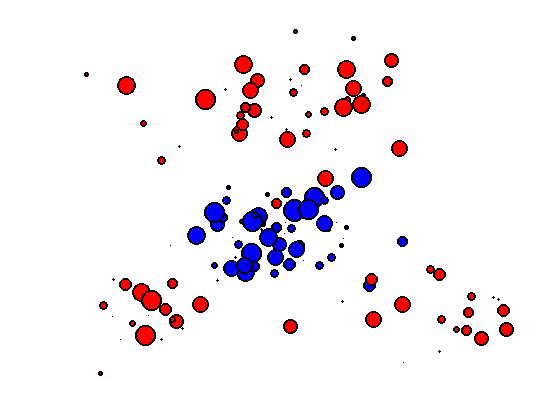Compute the weight matrix $$(C_{i,j})_{i,j}.$$

C = repmat( sum(X0.^2)', [1 n1] ) + ...
repmat( sum(X1.^2), [n0 1] ) - 2*X0'*X1;


Compute the optimal transport plan.

gamma = otransp(C,p0,p1);


Check that the number of non-zero entries in $$\ga^\star$$ is $$n_0+n_1-1$$.

fprintf('Number of non-zero: %d (n0+n1-1=%d)\n', full(sum(gamma(:)~=0)), n0+n1-1);

Number of non-zero: 139 (n0+n1-1=139)


Check that the solution satifies the constraints $$\ga \in \Cc$$.

fprintf('Constraints deviation (should be 0): %.2e, %.2e.\n', norm(sum(gamma,2)-p0(:)),  norm(sum(gamma,1)'-p1(:)));

Constraints deviation (should be 0): 9.03e-17, 6.13e-18.


## Displacement Interpolation

For any $$t \in [0,1]$$, one can define a distribution $$\mu_t$$ such that $$t \mapsto \mu_t$$ defines a geodesic for the Wasserstein metric.

Since the $$W_2$$ distance is a geodesic distance, this geodesic path solves the following variational problem $\mu_t = \uargmin{\mu} (1-t)W_2(\mu_0,\mu)^2 + t W_2(\mu_1,\mu)^2.$ This can be understood as a generalization of the usual Euclidean barycenter to barycenter of distribution. Indeed, in the case that $$\mu_k = \de_{x_k}$$, one has $$\mu_t=\de_{x_t}$$ where $$x_t = (1-t)x_0+t x_1$$.

Once the optimal coupling $$\ga^\star$$ has been computed, the interpolated distribution is obtained as $\mu_t = \sum_{i,j} \ga^\star_{i,j} \de_{(1-t)x_{0,i} + t x_{1,j}}.$

Find the $$i,j$$ with non-zero $$\ga_{i,j}^\star$$.

[I,J,gammaij] = find(gamma);


Display the evolution of $$\mu_t$$ for a varying value of $$t \in [0,1]$$.

clf;
tlist = linspace(0,1,6);
for i=1:length(tlist)
t=tlist(i);
Xt = (1-t)*X0(:,I) + t*X1(:,J);
subplot(2,3,i);
hold on;
for i=1:length(gammaij)
myplot(Xt(1,i), Xt(2,i), gammaij(i)*length(gammaij)*6, [t 0 1-t]);
end
title(['t=' num2str(t,2)]);
axis([min(X1(1,:)) max(X1(1,:)) min(X1(2,:)) max(X1(2,:))]); axis off;
end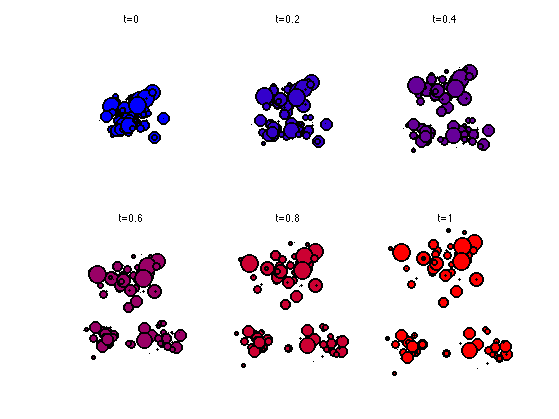## Optimal Assignement

In the case where the weights $$p_{0,i}=1/n, p_{1,i}=1/n$$ (where $$n_0=n_1=n$$) are constants, one can show that the optimal transport coupling is actually a permutation matrix. This properties comes from the fact that the extremal point of the polytope $$\Cc$$ are permutation matrices.

This means that there exists an optimal permutation $$\si^\star \in \Sigma_n$$ such that $\ga^\star_{i,j} = \choice{ 1 \qifq j=\si^\star(i), \\ 0 \quad\text{otherwise}. }$ where $$\Si_n$$ is the set of permutation (bijections) of $$\{1,\ldots,n\}$$.

This permutation thus solves the so-called optimal assignement problem $\si^\star \in \uargmin{\si \in \Sigma_n} \sum_{i} C_{i,\si(j)}.$

Same number of points.

n0 = 40;
n1 = n0;


Compute points clouds.

X0 = randn(2,n0)*.3;
X1 = [gauss(n1/2,.5, [0 1.6]) gauss(n1/4,.3, [-1 -1]) gauss(n1/4,.3, [1 -1])];


Constant distributions.

p0 = ones(n0,1)/n0;
p1 = ones(n1,1)/n1;


Compute the weight matrix $$(C_{i,j})_{i,j}.$$

C = repmat( sum(X0.^2)', [1 n1] ) + ...
repmat( sum(X1.^2), [n0 1] ) - 2*X0'*X1;


Display the coulds.

clf; hold on;
myplot(X0(1,:), X0(2,:), 10, 'b');
myplot(X1(1,:), X1(2,:), 10, 'r');
axis equal; axis off;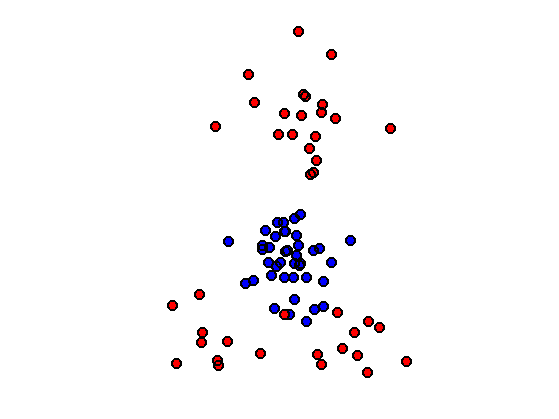Solve the optimal transport.

gamma = otransp(C,p0,p1);


Show that $$\ga$$ is a binary permutation matrix.

clf;
imageplot(gamma);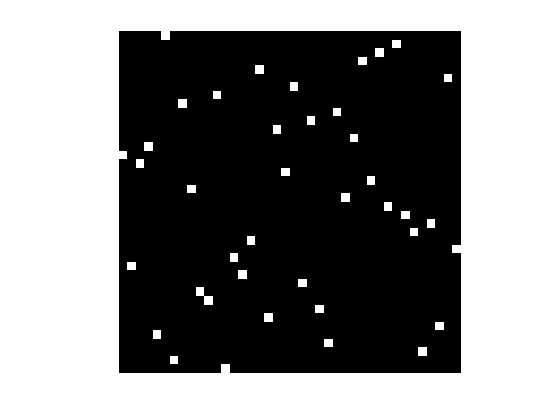Display the optimal assignement.

clf; hold on;
[I,J,~] = find(gamma);
for k=1:length(I)
h = plot( [X0(1,I(k)) X1(1,J(k))], [X0(2,I(k)) X1(2,J(k))], 'k' );
set(h, 'LineWidth', 2);
end
myplot(X0(1,:), X0(2,:), 10, 'b');
myplot(X1(1,:), X1(2,:), 10, 'r');
axis equal; axis off;